Home | Electronic | Fuzzy | Lecturer  | Power  | Transceiver

Find more type here...

Performance evaluation
of DC/AC inverter (2)

Seiichi Inoue

I will report the performance evaluation result of the inverter which was made this time.As the test bed, a battery for the car is used for the power supply and bulbs are used for the load. The internal resistance of the battery is very low and the big electric current (more over 100A) flows through the terminal when short-circuiting. So, you must not make short-circuit absolutely.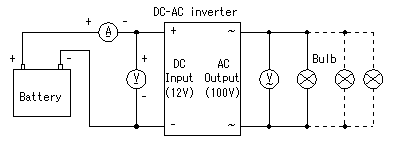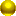The output power measurement

I measured the input voltage, the input electric current, the output voltage when changing the wattage of the bulb of the load. The output voltage falls when making a load big. The consumption electric power of the bulb changes with the voltage. So, I am converting to the consumption electric power according to the change of the voltage. In this case, I assume that the resistance value of the bulb doesn't change. However, the resistance value of the bulb changes with the consumption electric power. So, the result has errors by this conversion. Also, the voltage and the current are not sine wave. The result has more errors. However, I judged that these errors didn't have a big influence on the result.Ex. In case of the 60-W bulbThe resistance value of the bulb = V2/W = (100)2/60 = 167 ohms(I assume that this value doesn't change with the voltage.)The consumption electric power when the voltage is 98V = V2/R = (98)2/167 = 57.5 W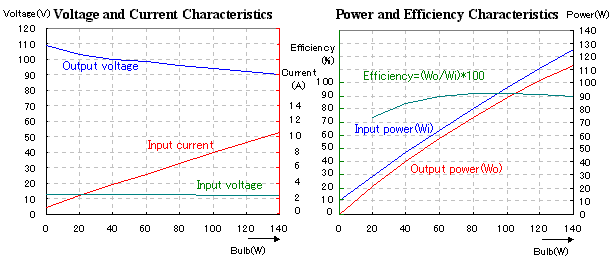When the output power is about 110W, the 10A electric current flows through the input. This is the maximun rating of this equipment. In this case, the output voltage is falling to about 90V. When connecting any more load, the output voltage falls more. So, in case of practical use, I think that the output of 120W is a limit of this equpment.The temperature rise measurement

I measured the temperature rise of the FET when an inverter is used in the continuous duty with the maximum load. The measurement place is the heat sink which the FETs are put on. I put a thermocouple thermometer to this part.The output voltage wave from

The output of this inverter is a square wave. When the load becomes big, the wave from of the output voltage will be slightly changed by the nature of a coil of the transformer.
There is to slightly change in the wave from of the rising edge and the falling edge. However, I think that it is permitted to say that it is changing hardly.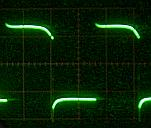No loaded60W loaded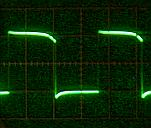110W loaded

This material comes from any source reference and the copyright is own the author him self not this sites